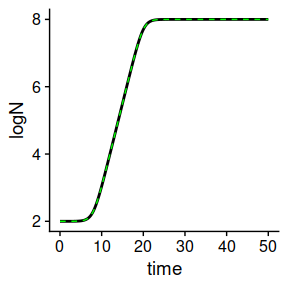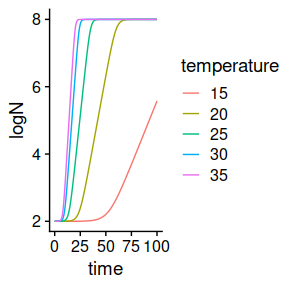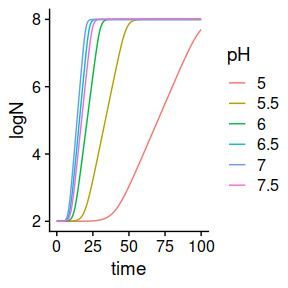# Using dynamic models for static environmental conditions

library(biogrowth)
library(tidyverse)
library(cowplot)

Although the function predict_dynamic_growth() is intended to describe growth under dynamic conditions, it can also be used for simulations under static conditions defining a constant environmental profile. This can be useful in situations were the environmental conditions are static, but the population response is defined using secondary models.

For starters, we will define an isothermal temperature profile at 35ºC.

my_conditions <- tibble(time = c(0, 50),
temperature = c(35, 35)
)

Next, we define primary and secondary models as usual.

q0 <- 1e-4
mu_opt <- .5

my_primary <- list(mu_opt = mu_opt,
Nmax = 1e8,N0 = 1e2,
Q0 = q0)

sec_temperature <- list(model = "CPM",
xmin = 5, xopt = 35, xmax = 40, n = 2)

my_secondary <- list(temperature = sec_temperature)

Finally, we call predict_dynamic_growth after defining the time points of the simulation.

my_times <- seq(0, 50, length = 1000)

## Do the simulation

dynamic_prediction <- predict_dynamic_growth(my_times,
my_conditions, my_primary,
my_secondary)

Because the temperature during the simulation equals the cardinal parameter $$X_{opt}$$, the predicted population size is identical to the one calculated using predict_isothermal_growth for the Baranyi model when $$\mu = \mu_{opt}$$ and $$\lambda = \frac{ \ln \left(1 +1/Q_0 \right) }{\mu_{opt}}$$.

lambda <- Q0_to_lambda(q0, mu_opt)

my_model <- "Baranyi"
my_pars <- list(logN0 = 2, logNmax = 8, mu = mu_opt, lambda = lambda)

static_prediction <- predict_isothermal_growth(my_model, my_times, my_pars)

plot(static_prediction) +
geom_line(aes(x = time, y = logN), linetype = 2, data = dynamic_prediction$simulation, colour = "green")The advantages of using predict_dynamic_growth() for modelling growth under static conditions are evident when simulations are made for several temperatures. Using predict_isothermal_growth() would require a calculation of the value of $$\mu$$ for each temperature separately. Because the relationship between $$\mu$$ and temperature is included in the secondary model, a separate calculation is not required when using predict_dynamic_growth(). max_time <- 100 c(15, 20, 25, 30, 35) %>% # Temperatures for the calculation set_names(., .) %>% map(., # Definition of constant temperature profile ~ tibble(time = c(0, max_time), temperature = c(., .)) ) %>% map(., # Growth simulation for each temperature ~ predict_dynamic_growth(seq(0, max_time, length = 1000), ., my_primary, my_secondary) ) %>% imap_dfr(., # Extract the simulation ~ mutate(.x$simulation, temperature = .y)
) %>%
ggplot() +
geom_line(aes(x = time, y = logN, colour = temperature)) +
theme_cowplot()Note, however, that predict_dynamic_growth() does not include any secondary model for the lag phase. The reason for this is that there are no broadly accepted secondary models for the lag phase in predictive microbiology. Therefore, the value of $$\lambda$$ varies among the simulations according to $$\lambda(T) = \frac{ \ln \left(1 +1/Q_0 \right) }{\mu(T)}$$.

Another application of predict_dynamic_growth() is including the impact of another environmental factor when temperature is kept constant. This can be done by defining a second secondary model.

my_primary <- list(mu_opt = mu_opt,
Nmax = 1e8,N0 = 1e2,
Q0 = q0)

sec_temperature <- list(model = "CPM",
xmin = 5, xopt = 35, xmax = 40, n = 2)

sec_pH <- list(model = "CPM",
xmin = 4, xopt = 7, xmax = 8, n = 2)

my_secondary_2 <- list(temperature = sec_temperature,
pH = sec_pH)

Then, we can call predict_dynamic_growth().

max_time <- 100

c(5, 5.5, 6, 6.5, 7, 7.5) %>%  # pH values for the calculation
set_names(., .) %>%
map(.,  # Definition of constant temperature profile
~ tibble(time = c(0, max_time),
temperature = c(35, 35),
pH = c(., .))
) %>%
map(.,  # Growth simulation for each temperature
~ predict_dynamic_growth(seq(0, max_time, length = 1000),
.,
my_primary,
my_secondary_2)
) %>%
imap_dfr(.,  # Extract the simulation
~  mutate(.x\$simulation, pH = .y)
) %>%
ggplot() +
geom_line(aes(x = time, y = logN, colour = pH)) +
theme_cowplot()As above, note that the lag phase varies between the simulations according to $$\lambda(T, pH) = \frac{ \ln \left(1 +1/Q_0 \right) }{\mu(T, pH)}$$.